Addition and Subtraction of Unlike Fractions

Chapter 7 Class 6 Fractions
Concept wise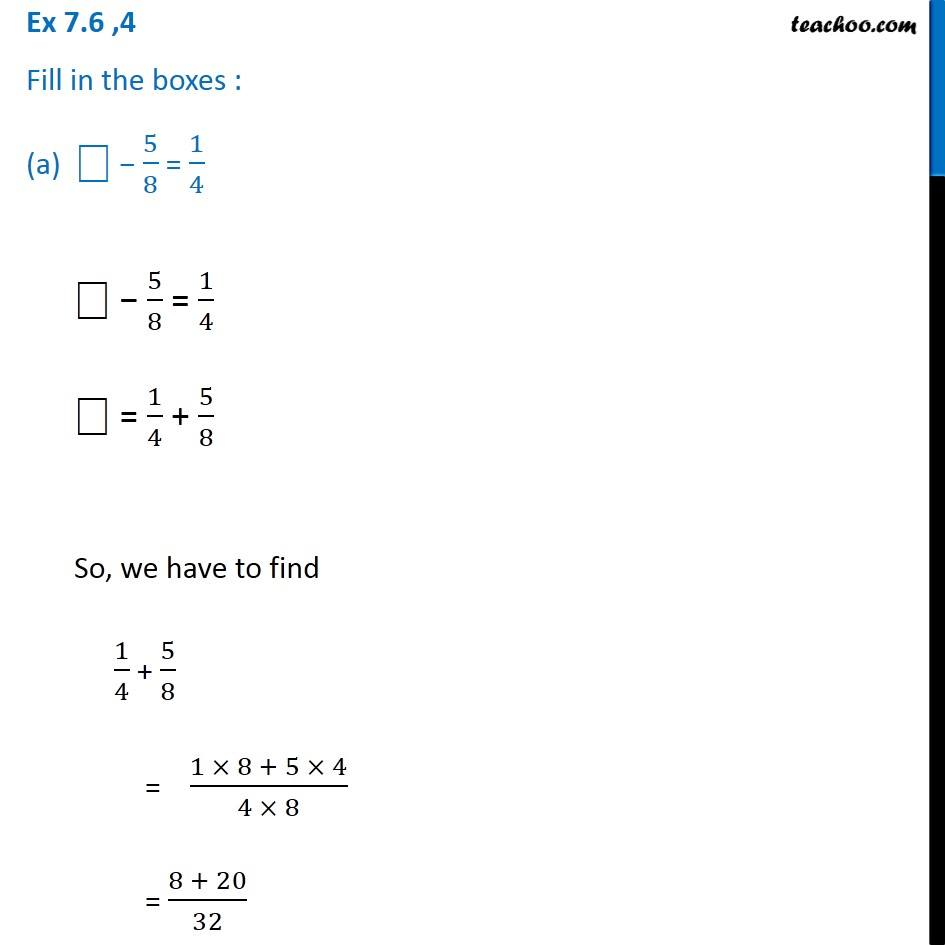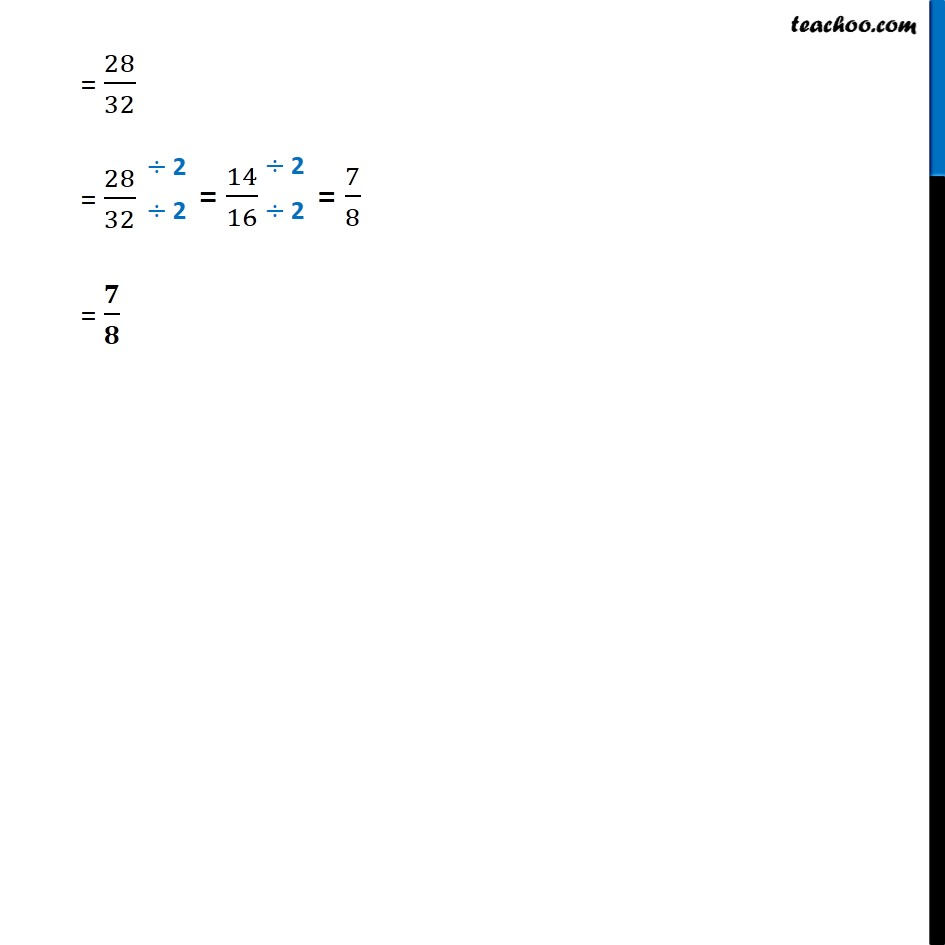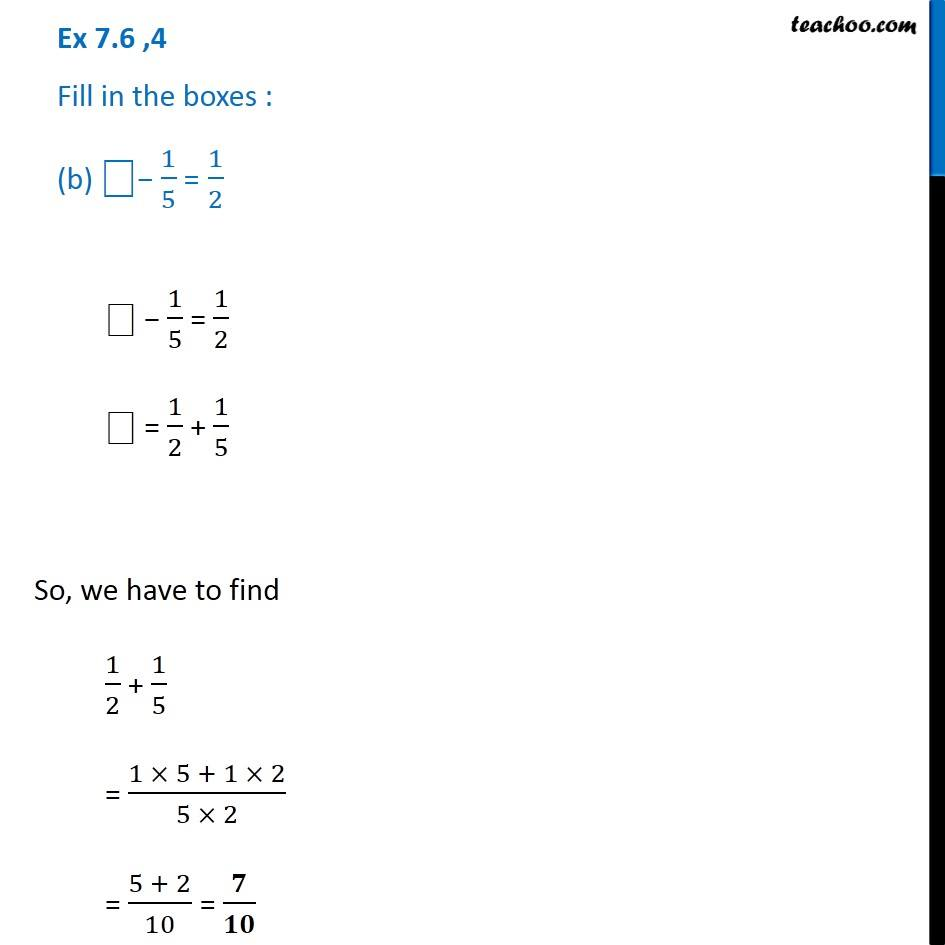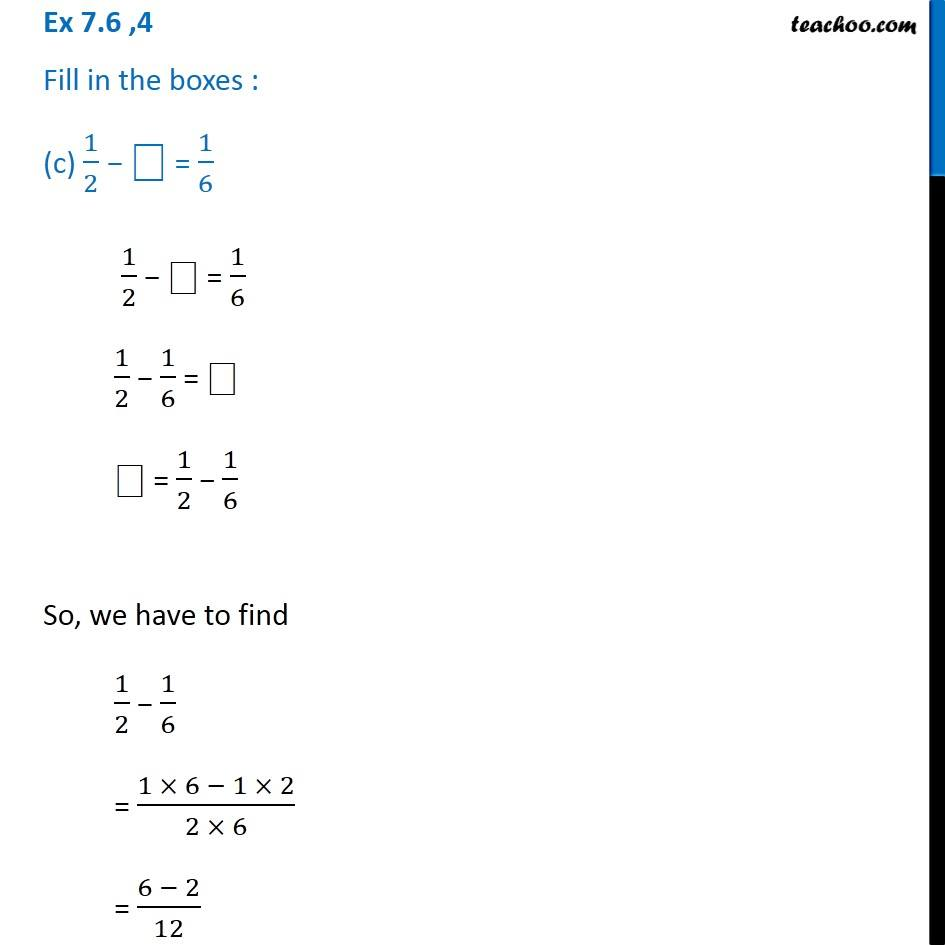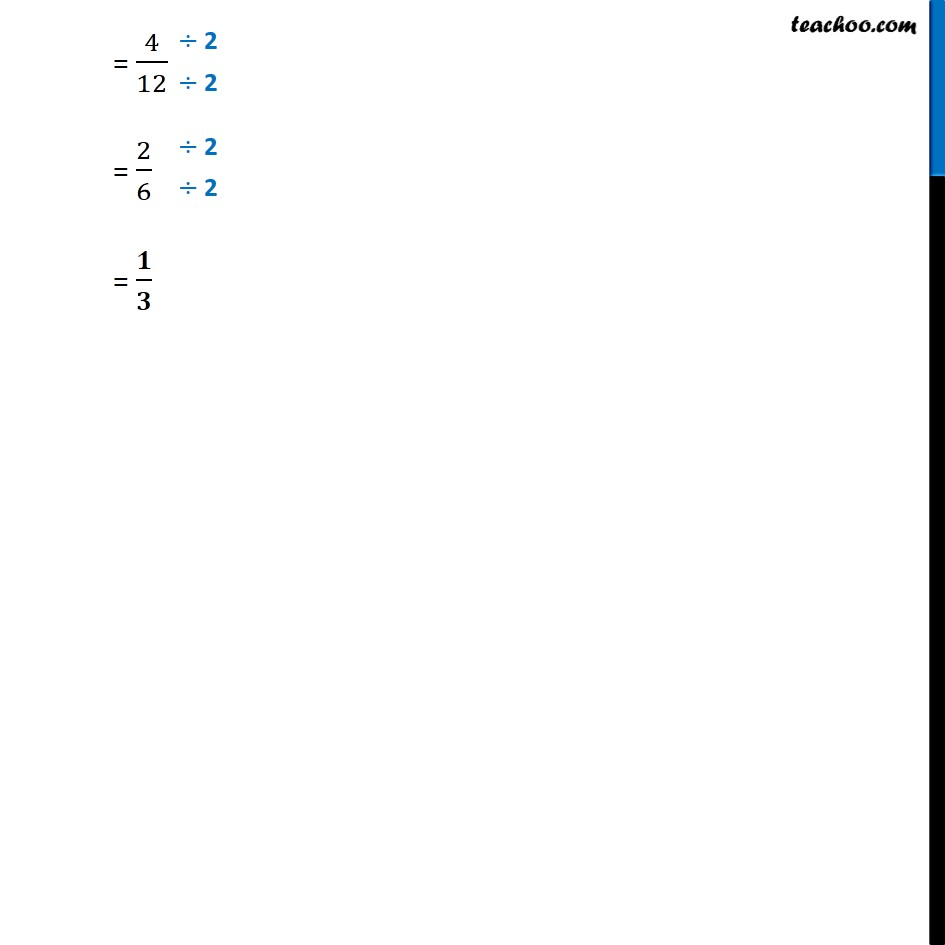Introducing your new favourite teacher - Teachoo Black, at only ₹83 per month

### Transcript

Ex 7.6 ,4 Fill in the boxes : (a) ⎕ − 5/8 = 1/4 ⎕ − 5/8 = 1/4 ⎕ = 1/4 + 5/8 So, we have to find 1/4 + 5/8 = (1 × 8 + 5 × 4)/(4 × 8) = (8 + 20)/32 = 28/32 = 28/32 = 𝟕/𝟖 Ex 7.6 ,4 Fill in the boxes : (c) 1/2 − ⎕ = 1/6 1/2 − ⎕ = 1/6 1/2 − 1/6 = ⎕ ⎕ = 1/2 − 1/6 So, we have to find 1/2 − 1/6 = (1 × 6 − 1 × 2)/(2 × 6) = (6 − 2)/12 = 4/12 = 2/6 = 𝟏/𝟑